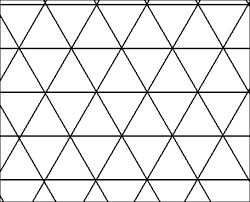# Schläfli symbols

What is the dual of an infinite triangular lattice?Definitions:

• The dual of a solid with Schläfli symbols $\{m,n\}$ has Schläfli symbols $\{n,m\}.$

• The Schläfli symbols for a geometric construct is defined by $\{m,n\}$, where $m$ is the number of edges for each face and $n$ is the number of faces that meet at a vertex.

×